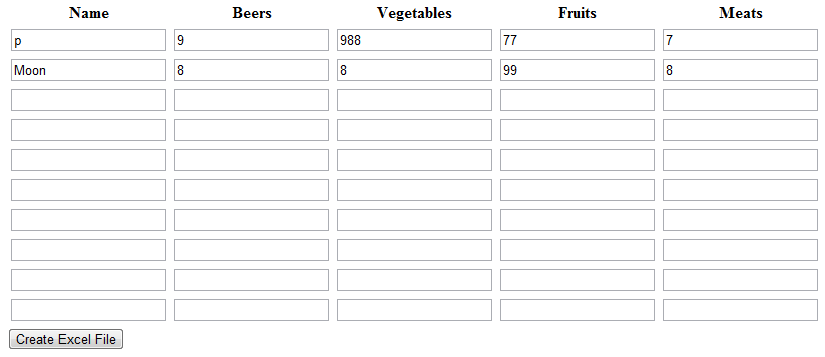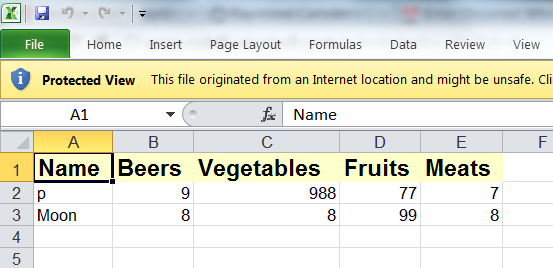In this ColdFusion sample, I'll demonstrate a simple way to take form input and generate an Excel spreadsheet file. This is a simple example with a very basic form, but hopefully it will give you enough to go by to create your own similar application.

Let's begin by creating a simple form. This form is going to ask for 10 rows of data that mimics the Excel sheet I uploaded in my previous blog entry. It will ask for a name and the number of beers, vegetables, fruits, and meats consumed.

`<form action="test2.cfm" method="post"> <table> <tr> <th>Name</th> <th>Beers</th> <th>Vegetables</th> <th>Fruits</th> <th>Meats</th> </tr> <cfloop index="x" from="1" to="10"> <cfoutput> <tr> <td><input type="text" name="name_#x#"></td> <td><input type="text" name="beers_#x#"></td> <td><input type="text" name="veggies_#x#"></td> <td><input type="text" name="fruits_#x#"></td> <td><input type="text" name="meats_#x#"></td> </tr> </cfoutput> </cfloop> </table> <input type="submit" name="doit" value="Create Excel File"> </form>`

This form could be more dynamic of course. You could present 3 rows and use jQuery (or another less capable JavaScript framework) to easily add additional rows for input. But to keep things simple the form will just create 10 rows. Here's how it looks - and again - more work could be done to make this friendlier to the end user.Ok - now to generate the spreadsheet. Let's look at an example.

`<cfset q = queryNew("Name,Beers,Vegetables,Fruits,Meats", "cf_sql_varchar,cf_sql_integer,cf_sql_integer,cf_sql_integer,cf_sql_integer")> <cfloop index="x" from="1" to="10"> <cfset queryAddRow(q)> <cfset querySetCell(q, "Name", form["name_#x#"])> <cfset querySetCell(q, "Beers", form["beers_#x#"])> <cfset querySetCell(q, "Vegetables", form["veggies_#x#"])> <cfset querySetCell(q, "Fruits", form["fruits_#x#"])> <cfset querySetCell(q, "Meats", form["meats_#x#"])> </cfloop>`

`<cfset filename = expandPath("./myexcel.xls")> <cfspreadsheet action="write" query="q" filename="#filename#" overwrite="true">`

We begin by turning the form data into a query. This is done by looping from 1 to 10 and grabbing the relevant values from the Form struct. You could do a trim and other checks here as well. The real magic of this code block is the last line. All it takes to create a spreadsheet in ColdFusion is one line. You pass in the query, filename, and... that's it! While this works, the output is a bit plain. Let's now look at a slight modification.

`<!--- Make a spreadsheet object ---> <cfset s = spreadsheetNew()> <!--- Add header row ---> <cfset spreadsheetAddRow(s, "Name,Beers,Vegetables,Fruits,Meats")> <!--- format header ---> <cfset spreadsheetFormatRow(s, { bold=true, fgcolor="lemon_chiffon", fontsize=14 }, 1)>`

`<!--- Add query ---> <cfset spreadsheetAddRows(s, q)> <cfset spreadsheetWrite(s, filename, true)>`

This version is a bit more complex. We begin creating a blank spreadsheet object. Next we add the header row. We can format both cells and rows, and in this example I've gone ahead and formatted the header. You have many options but I used just bold, fgcolor, and fontsize. (No one make any comments about lemon chiffon, it rocks.)

Next I add the data using spreadsheetAddRows and write out the data. Here's a quick example of the result.Here's the entire code template.

`<cfif not structKeyExists(form, "doit")>`

`<form action="test2.cfm" method="post"> <table> <tr> <th>Name</th> <th>Beers</th> <th>Vegetables</th> <th>Fruits</th> <th>Meats</th> </tr> <cfloop index="x" from="1" to="10"> <cfoutput> <tr> <td><input type="text" name="name_#x#"></td> <td><input type="text" name="beers_#x#"></td> <td><input type="text" name="veggies_#x#"></td> <td><input type="text" name="fruits_#x#"></td> <td><input type="text" name="meats_#x#"></td> </tr> </cfoutput> </cfloop> </table> <input type="submit" name="doit" value="Create Excel File"> </form>`

`<cfelse>`

`<cfset q = queryNew("Name,Beers,Vegetables,Fruits,Meats", "cf_sql_varchar,cf_sql_integer,cf_sql_integer,cf_sql_integer,cf_sql_integer")> <cfloop index="x" from="1" to="10"> <cfset queryAddRow(q)> <cfset querySetCell(q, "Name", form["name_#x#"])> <cfset querySetCell(q, "Beers", form["beers_#x#"])> <cfset querySetCell(q, "Vegetables", form["veggies_#x#"])> <cfset querySetCell(q, "Fruits", form["fruits_#x#"])> <cfset querySetCell(q, "Meats", form["meats_#x#"])> </cfloop>`

`<cfset filename = expandPath("./myexcel.xls")> <!--- <cfspreadsheet action="write" query="q" filename="#filename#" overwrite="true"> ---> <!--- Make a spreadsheet object ---> <cfset s = spreadsheetNew()> <!--- Add header row ---> <cfset spreadsheetAddRow(s, "Name,Beers,Vegetables,Fruits,Meats")> <!--- format header ---> <cfset spreadsheetFormatRow(s, { bold=true, fgcolor="lemon_chiffon", fontsize=14 }, 1)>`

`<!--- Add query ---> <cfset spreadsheetAddRows(s, q)> <cfset spreadsheetWrite(s, filename, true)>`

`Your spreadsheet is ready. You may download it <a href="myexcel.xls">here</a>.`

`</cfif>`